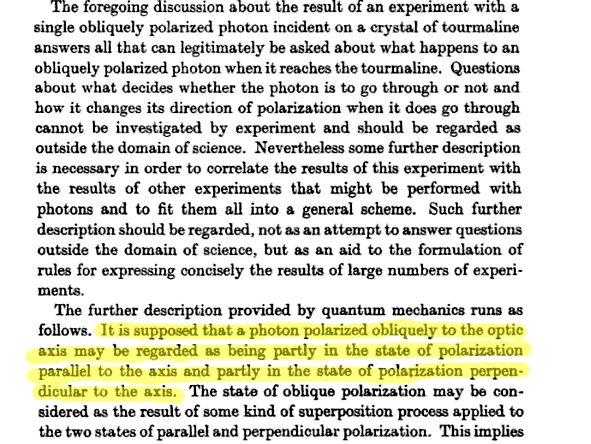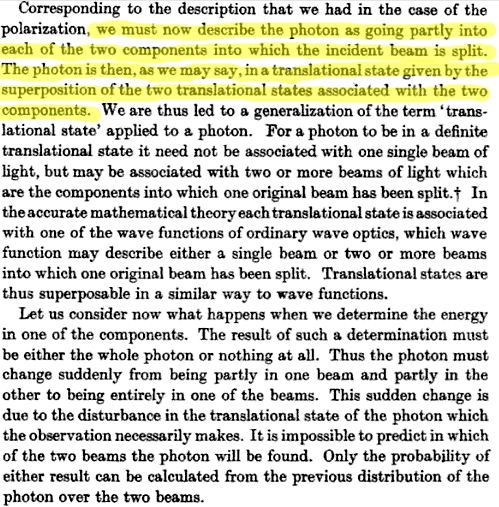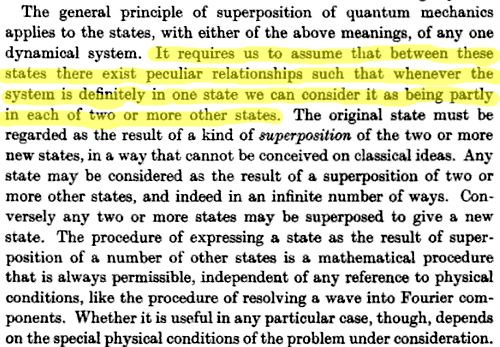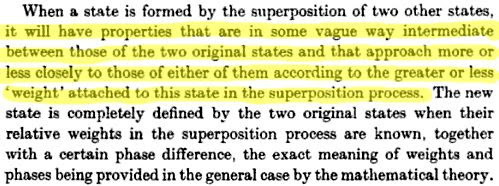# Problem in understanding the concept of 'superposition' as explained by Dirac

The concept of 'superposition' has really made me insane, actually. What I thought it was just simple superposition of matter waves. For instance, let's take the Double-Slit experiment: take the source as $S.$ The two slits are $1$ & $2.$

\begin{align}\psi_S(x) = \text{probability amplitude of finding the particle at} \;x\; \text{given that it originated at the source} \;S\\ \psi_S(n) \psi_n(x) = \text{Probability amplitude of observing the particle at slit} \;n\; \text{given that it originated from the source} \;S. \;\times\; \text{Probability amplitude of observing the particle at}\; x\;\text{given that it originated from slit} \;n. \\= \text{Probability amplitude for the particle to pass from the source}\; S\; \text{to point}\; x\; \text{through slit}\; n.\end{align}

Therefore, $$\psi_S(x) =\langle x|S\rangle\\ \&\\ \psi_S(x)= \psi_S(1) \psi_1(x)+\psi_S(2) \psi_2(x)\\ \implies \langle x|S\rangle= \langle x|1\rangle\langle1|S\rangle + \langle x|2\rangle\langle 2|S\rangle.$$

What does that mean?

To me, this wave function will propagate through space and at the positions of the two slits. These waves(not real, but of mathematical construct: the probability wave) will then pass through these slits on their way towards the observation screen. The amplitude of the waves at a point $x$ on the observation screen is due to the superposition of waves that originated at each of the slits. The amplitudes of the probability waves superpose with each other like the superposition of classical waves that is, $\langle x|1\rangle\langle1|S\rangle + \langle x|2\rangle\langle 2|S\rangle.$ So, the concept of superposition was to me as the superposition of probability waves.

What does this then mean? $$|S\rangle= |1\rangle\langle1|S\rangle + |2\rangle\langle 2|S\rangle.\tag{7.12}$$

Till now, I bore the concept of the state $|S\rangle$ being in superposition of $|1\rangle$ & $|2\rangle$ that remaining in state $|S\rangle$ means there is a possibility to be in $|1\rangle$ & also in $|2\rangle.$ It is like the disjoint mutually exclusive events in classical theory which states if _an event $S$ can occur in more than one way which are mutually exclusive, say $1$ & $2$, then the probability of occurance of that event $S$ is given by $P(S)= P(1) + P(2).$ What does this mean? That $S$ can happen by either $1$ or $2$ but they don't happen simultaneously. Same is in the quantum case, but this time, it is of amplitude & not probability that being in state $S$ mean you can be either at state $1$ or $2$ with the coefficients giving the amplitudes. I never could take that superposition means to be in both $1$ & $2$ simultaneously. I thought always the superposition as the superposition of the amplitudes of the probability waves to be at $1$ & $2$.

But now, after reading The principles of Quantum Mechanics by P.A.M Dirac, I am quite dubious of my concept:What does Dirac mean by 'partly' in this state & 'partly' in another state? Is one part of the photon in parallel-polarization & other part in the other type? How can one part be at one type of polarization & other part of the same photon in another polarization?

In another example, he writes,Now, the photon is divided into two beams! One part goes into one beam & another part goes of the same photon has gone to another beam! But I knew, photon cannot be split (of course, if the energy is same, a single photon can be split, but here, it is is not the same).

Ultimately, Dirac defines precisely what superposition is:So, according to him, superposition means partly in one state & partly in another state. But what does partly mean? Does the photon get divided so that its one part is at one state & other part is at another state?

I'm really not getting what Dirac wants to mean by partly.

Let's take an electron & base states as different coordinates along the $x$-axis. So, $|x_1\rangle, |x_2\rangle, |x_3\rangle \ldots$ are the base states. If I make a state $$|\psi\rangle= \sum_i |x_i\rangle c_i$$, which implies $|\psi\rangle$ is in superposition, does that mean that the electron is partly at $x_1$, partly at $x_2$, partly at $x_3$ & so on? Does that mean the electron get parted?

In Does electron being many places at the same time violate Physics laws?, @anna v completely disgraces the fact that electron can be at many places at a single time. But it is written by Dirac, one of the pioneers of QM, that superposition means partly in one state, partly at other one. But if I apply it as above it yields contradictory result that electron can be at many places at the same time but that is totally nonsense, isn't it?

The new interpretation is a very potent one, and constitutes the central feature of quantum mechanics,the idea that a system, in some sense, can be simultaneously in a number of different physical states, otherwise known as a ‘superposition of states’.

[...] Put another way, the expression Eq. $(7.12)$ indicates in a symbolic way the fact that when the particle is prepared in its initial state $|S\rangle,$ there is built into this state the potential of the particle being found in the other states $|n\rangle,$ with the chances of the particle being found in these other states being given by the coefficients & $\langle n|S\rangle.$ It is this sort of result that lies at the heart of quantum mechanics – the idea that a system in a certain state can behave as if it is in one or another of a number of other states, and to do so in a way that is probabilistic in nature.

Here, the author at first uses 'in some sense'; in the next paragraph, he uses the word potential of the particle being found in other states simultaneously & lastly the line and to do so in a way that is probabilistic in nature. What does he mean by the last line? Does potential mean the same as partly?

What does superposition actually mean? To be partly at one state & partly at other state, is it so? But that seems to be contradictory:( What does it mean to be 'built into this state the potential of the particle being found in the other states'?

Really, could anyone please help me understand the concept of superposition, the explanation of Dirac to be partly in one state & partly at other?

[N.B.: $\bullet$ Sorry for posting pics of Dirac's pages, but I couldn't type all these as they can't be copied from google books.

$\bullet$ What does superposition mean in quantum mechanics? though seems to be related is not at all a duplicate of this question. In that question, OP has asked about what superposition meant; I also want to know about that but there it was not in the context of Dirac's language. Also, there OP was ignorant of the fact; but here I have the conception which seems to be contradicting with that of Dirac or the other linked source; I want to know that reason.]

# Edit:

After getting a quite intuitive answer from @ACuriousMind, I am adding another part of Dirac's explanation that I need to get the nerve:What did he mean by that? So, does that mean an electron being in the state $|S\rangle$ mean that the electron has properties intermediate of the properties when being at $|1\rangle$ & $|2\rangle$? I'm not quite getting the point what he meant by that; being at $|s\rangle$ means the electron is at position $S$; how could it have property intermediate of $|1\rangle$ & $|2\rangle$? Also, why does Dirac refer the coefficients as weight? What does he mean by that? I thought these coefficients refer the amplitude of finding the electron at slit $1,2$ provided it has originated at $S.$ I really am not getting what he meant by that term.

Can anyone please explain the marked statement of Dirac with some example?

The phrase

Superposition of quantum states means that a state is partly in one state and partly in another

is correct, but only if we know what we mean by "partly". If there are states $\lvert n \rangle$ that we are able to measure (as the eigenstates of an observable (self-adjoint operator) $N$), these states span every vector in the Hilbert space of states by the spectral theorem, i.e. every $\lvert \psi \rangle$ can be written as the superposition of these states: $$\lvert \psi \rangle = \sum_n c_n\lvert n \rangle$$ By the Born rule, the probability to measure $n$ for the value of $N$ is given by $$\frac{\lvert\langle \psi \vert n \rangle\rvert^2}{\langle \psi \vert \psi \rangle \langle n \vert n \rangle}$$ which is just $\lvert c_n \rvert^2$ if the states are normalised. If more than one $c_n$ is non-zero, we say that the thing is partly in these states.

Does that mean the electron get parted?

or, in this case, is the particle in the state $\lvert \psi \rangle$ parted into a part of it in state $\lvert n_1 \rangle$, another in $\lvert n_2 \rangle$, and so on.

Well, no. It isn't a classical particle to begin with. It's a quantum object that is fully characterized by its quantum state, and nothing else. It doesn't make sense to speak of its position as a point if it is not in a position eigenstate, and it doesn't make sense to ask if it gets "parted". What your quotes mean by "partly" is that, sometimes, you'll see it in the one state when you measure, and sometimes you'll see it in the other. This could be construed as "being in both places at once" prior to measurement, but this "being" is not the classical existence I think you're imagining. There are no "parts" of the electron flying along the possible paths in any classical sense, because the classical idea of a (point or extended) classical particle just doesn't work in the quantum treatment of physics.

• You may be right, sir, in your statement but this "being" is not the classical existence I think you're imagining. But then what 'being' are you a actually talking of? I thought, BTW, that the particle has the possibility to exist at different places; I know it is not a classical particle but can you please make it a bit clearer what a quantum particle or entity actually is if it is not a classical particle? Thanks for the help, sir.
– user36790
Oct 8 '15 at 19:19
• @user36790: Well, if you like it better, you can also say that it has the "possibility to exist" in the states it "is partly in". Since all that physically counts is the probability distribution of the measurement results, it doesn't make a difference. The quantum object is just that what is described by the state. We don't know anything more about it, and we don't need to to get the physics. Oct 8 '15 at 19:35
• Sir, I've added another part of my query; can you please check that?
– user36790
Oct 9 '15 at 14:26
• @ACuriousMind Hi there mate, fantastic answer, thanks for the clarifications. Oct 15 '15 at 11:48

"Superposition" means exactly the same thing as "linear combination". When the state of a particle is a linear combination of states B and C, Dirac, speaking loosely, says that it is "partly in state B and partly in state C". This kind of loose talk is not meant to be taken terribly seriously.